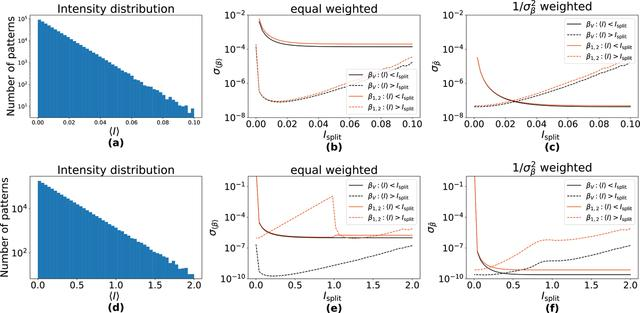disable zoom     view article Figure 5 Effects of-weighting demonstrated on simulated data in which the mean counts per pattern follows exponential distributions with (a) mean= 0.01 and (d)= 0.2. The data were divided into two parts: one at high intensity with 〈I〉 > Isplit, and its complement with 〈I〉 ≤ Isplit. (b, e) Standard deviation of the retrieved β of the two parts, using equal weighting, as a function of Isplit. The standard deviation decreases when neglecting the patterns with very low counts as evident in the low-intensity regime of (b). The plot ofin (e) exhibits a sharp discontinuity at Isplit = 1, which is absent for. (c, f) Standard deviation of the retrieved β of the two parts, using-weighting. In this case the lowest standard deviation is achieved by using all patterns to estimate β. βV always performs better than β1, 2, especially in the high-intensity regime, see (f).JOURNAL OFSYNCHROTRONRADIATION
ISSN: 1600-5775
Volume 30| Part 1| January 2023| Pages 11-23